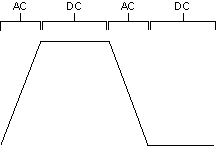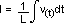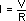From 9:00 AM - 12:00 PM CDT on Saturday, June 6th, ni.com will be undergoing system upgrades that may result in temporary service interruption.

We appreciate your patience as we improve our online experience.

# AC and DC Current

NI Digital Waveform Generator/Analyzer Help

Edition Date: June 2013

Part Number: 370520P-01

»View Product InfoDownload Help (Windows Only)

AC current is current sourced/sunk during the transition between low and high level states driving a capacitive load (such as a cable) or an inductive load (such as a device interconnect). DC current is the current sourced/sunk when the generation terminals are at a static voltage driving a resistive load. The following illustration of a digital waveform shows when AC or DC current is sourced/sunk.Use the following formulas to calculate AC current:where    I is current in amps,

dV/dt is the rate of change of the voltage level in volts/s

orwhere    I is the current in amps

V(t) is the voltage in volts as a function of time

Use the following formula to calculate DC current:where    I is current in amps

V is voltage level in volts

R is resistance in ohms